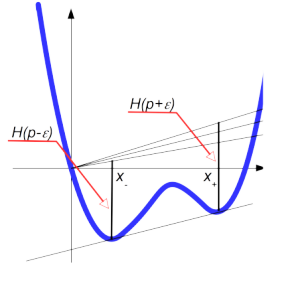ECE 515, week of Dec. 3

$$\def\Real{\mathbb{R}}$$

• Pontryagin maximum principle
• Examples
• Lagrangians to Hamiltonians: Fenchel-Legendre transform.

Legendre (sometimes called Legendre-Fenchel) transform $$H$$ of a convex function $$L:\Real\to\Real$$) is defined as
$H(p):=\sup_x px-L(x).$
Both functions, $$L$$ and $$H$$ can take value $$+\infty$$.

If $$L$$ is strictly convex, the gradient mapping
$G:x\mapsto \frac{dL}{dx}$
is one-to-one, and it is clear that the argmax in the definition of $$H$$ solves $$p=G(x)$$. Denote the functional inverse to $$G$$ as $$F: p=G(x) \Leftrightarrow x=F(p)$$. In this case,
$H(p)=pF(p)-L(F(p)).$
Differentiating, we obtain
$\frac{dH}{dp}=F(p)+\left(p-\frac{dL}{dx}\right)\frac{dF}{dp}=F(p)$

Hence the graph of the derivative of $$L$$ with respect to $$x$$, in the $$(x,p)$$ space is the same as the graph of the derivative of $$H$$ with respect to $$p$$. It follows immediately that the Legendre transform is involutive:
$L(x)=\sup_p px-H(p).$

If $$L$$ is convex, one can turn it into a strictly convex function by adding $$\epsilon |x|^2/2$$. The graph of the resulting gradient,
$p=G(x)+\epsilon x$
converges to the graph of $$p=G(x)$$ as $$\epsilon \to 0$$. So one can define the graph $$p=G(x)$$ for all convex functions.

One can also define Legendre transform for nonconvex $$L$$, using the same formula. The correspondence won’t involutive anymore (as Legendre-Fenchel transforms, as defined above, are always convex). Still, one can reproduce many results, in particular, that the derivative of $$H$$ is discontinuous at $$p$$ iff $$L-px$$ has two competing global maxima, see figure below.Increments of $$H$$ near $$p$$ are and $$x_-$$ and $$x_+$$, respectively: the function $$H$$ is not continuously differentiable.

These considerations are relevant for the Homework 5, as in Problem a,
$V(x,0)=\inf_X \frac{(x-X)^2}{2T}+\cos(X)=\frac{x^2}{2T}-\frac{1}{T} \sup \left(xX-\left(\frac{X^2}{2}+T\cos(X)\right)\right),$
i.e. is (somewhat modified) Legendre transform of the function $$X^2+T\cos X$$.

For more, see chapter on Legendre transform in Arnold’s Mechanics, p 61ff.

• Transversality: boundary conditions as Lagrangian manifolds
• Minimal time linear problems. Bang-bang control.
• Sketch of the proof of PMP.

4 Responses to ECE 515, week of Dec. 3

1. Jasvir Virdi December 8, 2018 at 9:54 am #

Does this result imply that for a non convex function have 2 competing global maxima/minima, the function will be not continuously differentiable?

• yuliy December 9, 2018 at 5:38 pm #

Yes, if px-L has two competing maxima, H is not smooth at p.

2. Anthony S Podkowa December 9, 2018 at 5:09 pm #

In the last equation, is cos(T) supposed to be cos(x(T))=cos(X)? I don’t see how it is possible to extract the T out. Please let me know.

• yuliy December 9, 2018 at 5:39 pm #

Thank, Tony, – typo corrected.